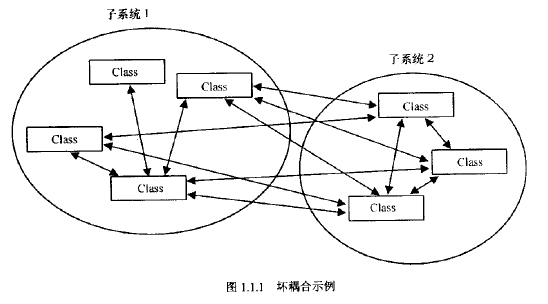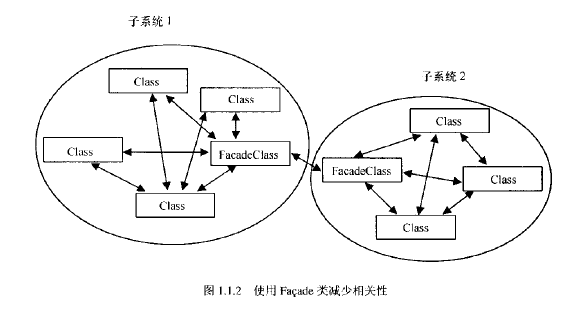# 游戏编程精粹1

## 第一章：通用编程技术

Posted by LudoArt on March 1, 2021

# 1.1 面向对象的编程与设计技术

## 1.1.2 类设计

``````class Sample
{
public:
Sample()	{ Clear(); }
~Sample()	{ Destroy(); }

void Clear();

bool Create();
void Update();
void Destroy();
};
``````

`Clear()`函数用于清空所有内部成员变量。

`Create()`函数有两种返回值可以选择：一种是简单的布尔值表示；另一种是使用标准的错误代码类型，通常为符号整型。

`Destroy()`函数，因为我们既希望有自动清空的便利，又希望有“按需创建和删除”的灵活性，所以我们需要确保`Destroy()`函数能安全地多次调用，或在没有调用`Create()`函数的情况下也能安全调用。记住在销毁函数的最后，一定要调用`Clear()`函数来将所有对象重置回初始状态。

## 1.1.3 类层次结构设计

• 继承就是从一个类派生出另一个类；
• 分层则是指一个对象作为成员包含于另一个对象，也称作组合、容器与嵌套。

## 1.1.4 设计模式

1. Singleton（单件）模式

当大量的类和/或模块需要访问一个全局对象时，使用Singleton模型。Singleton强迫通过一个类来进行访问，这个类中存储有一个内部静态对象。以下是大致的基本实现形式：

``````class Singleton1
{
public:
Singleton1& Instance()
{
static Singleton Obj;
return Obj;
}
private:
Singleton1();
};
``````

如果要从它派生出新的类，可以改变设计：

``````// singleton基类
class SingletonBase()
{
public:
SingletonBase()
{ cout << "SingletonBase created!" << endl; }
virtual ~SingletonBase()
{ cout << "SingletonBase destroyed!" << endl; }
virtual void Access()
{ cout << "SingletonBase accessed!" << endl; }
static SingletonBase* GetObj()
{ return m_pObj; }
static void SetObj(SingletonBase* pObj)
{ m_pObj = pObj; }
protected:
static SingletonBase* m_pObj;
};

SingletonBase* SingletonBase::m_pObj;

inline SingletonBase* Base()
{
assert(SingletonBase::GetObj());
return SingletonBase::GetObj();
}

// 创建一个派生的singleton类型
class SingletonDerived : public SingletonBase
{
public:
SingletonDerived()
{ cout << "SingletonDerived created!" << endl; }
virtual ~SingletonDerived()
{ cout << "SingletonDerived destroyed!" << endl; }
virtual void Access()
{ cout << "SingletonDerived accessed!" << endl; }
protected:
};

inline SingletonDerived* Derived()
{
assert(SingletonDerived::GetObj());
return (SingletonDerived*)SingletonDerived::GetObj();
}

// 使用代码
// 复杂的singleton的使用需要做更多工作，而这个更加灵活
// 它还允许对对象创建进行更多的控制，这有时候非常有用
SingletonDerived::SetObj(new SingletonDerived);

// 注意这个方法的功能被新类重载了
// 即使通过原有方法访问也是如此
Base()->Access();
Derived()->Access();

// 很不幸，singleton上的这个变量要求显示地创建和销毁
delete SingletonDerived::GetObj();

``````

Scott Bilas所写的《一种自动的singleton工具》文章中提出了对singleton模式的另一种变体，他以一种优雅的方式使用模板和公有继承来自动创建singleton类。3. State（状态）模式

使用对象的好处是：状态得到了更好的封装，状态可以在它们的基类中逻辑地分享代码，且新的状态可以通过继承轻易地从现有状态中派生出来。 这些好处减少了再离散的状态间进行剪切、粘贴代码所带来的典型问题。

采用一个中央管理器来处理这些类的过渡，可以避免内部对象之间的关联性，只需要管理器知道所有不同的状态对象就可以了。

4. Factory（工厂）模式

Factory模式用于组织对象的创建，它的一种形式定义为一种方法，允许抽象接口类指定何时创建具体的派生实现类。

把对象分配聚集到一个位置的好处：

• 因为动态内存分配很昂贵，在一个中央区域分配所有的对象使得分配监控变得更为容易。
• 通常，全体对象的公共初始化或创建方法应该在一个类层次结构中。如果把对全体对象的分配放到一个中央区域，就使得基于全体对象的操作变得容易了。
• 工厂具有可扩充性，它允许新的对象从现有工厂中派生出来。通过传递新的类ID，就可以在运行时扩充新的类而不用改变现有的基代码。
``````BaseClass* ClassFactory::CreateObject(int id)
{
BaseClass* pClass = 0;
switch(id)
{
case 1:
pClass = new Class1;
break;
case 2:
pClass = new Class2;
break;
case 3:
pClass = new Class3;
break;
default:
assert(!"Error!");
};

pClass->Init(); // 也许需要进行一些一般性的初始化工作

return pClass;
}
``````

当在一个对象层次结构中的大量对象需要在运行时动态创建的时候，就可以使用工厂模式，这些对象可以包括AI对象、资源或者更抽象的对象。

## 1.1.5 总结与参考资料

Gamma, et.al., Design Patterns, Addison-Wesley Longman, Inc., 1994.

Meyers, Scott, Effective C++, second edition, Addison-Wesley Longman, Inc., 1996.

Petzold, Charles, Programming Windows 95, Microsoft Press, Inc., 1996.

# 1.2 使用模板元编程的快速数学方法

## 1.2.1 斐波那契数

``````unsigned RecursiveFib(unsigned n)
{
if(n <= 1)
return n;
return RecursiveFib(n - 1) + RecursiveFib(n - 2);
}
``````

``````template<unsigned N> struct Fib
{
enum
{
// 递归定义
Val = Fib<N-1>::Val + Fib<N-2>::Val
};
};

// 基本情况的模板特殊（结束条件）
template <> struct Fib<0> { enum { Val = 0 }; };
template <> struct Fib<1> { enum { Val = 1 }; };

// 让该模板形如函数
#define FibT(n) Fib<n>::Val

// 通过#define“调用”此模板
std::cout << FibT(4); // Fib<4>::Val
``````

• 模板函数并不是真正的函数——它是叫做Val的枚举整数，在编译期递归生成。
• Fib被定义为结构，以简化标记。在默认情况下结构数据是公用的。
• 模板参数N用于指定函数的输入。
• 要中止递归，需要正确地处理结束条件。在模板中处理基本情况的方法是使用模板特化。

## 1.2.2 阶乘

``````unsigned RecursiveFact(unsigned n)
{
return ((n <= 1) ? 1 : (n * RecursiveFact(n - 1)));
}
``````

``````// 模板化阶乘
template< unsigned N > struct Fact
{
enum { Val = N * Fact< N - 1 >::Val };
};

// 基本情况的模板特化
template < > struct Fact< 1 >
{
enum { Val = 1 };
};

// 让模板形如函数
#define FactT(n) Fact< n >::Val
``````

## 1.2.3 三角学

``````double Sine(double fRad)
{
const int iMaxTerms = 10;
}

double SineSeries(double fRad, int i, int iMaxTerms);
{
if(i > iMaxTerms)
return 1.0;

return 1.0 - (fRad * fRad / (2.0 * i + 2.0) / (2.0 * i + 3.0) * SineSeries(fRad, i + 1, iMaxTerms));
}
``````

``````// sin(R)级数展开
// 对符合标准的编译器，将double R改为double& R
template<double R> struct Sine
{
enum { MaxTerms = 10 }; // 增加精确性
static inline double sin()
{
return R * Series<R, 0, MaxTerms>::val();
}
};

template<double R, int I, int MaxTerms>
struct Series
{
enum
{
// Continue为true，知道我们已经计算了M项
Continue = I + 1 != MaxTerms,
NxtI = (I + 1) * Continue,
NxtMaxTerms = MaxTerms * Continue;
};

// 递归定义，
static inline double val()
{
return 1 - R * R / (2.0 * I + 2.0) /
(2.0 * I + 3.0) * Series<R * Continue, NxtI, NxtMaxTerms>::val();
}
};

// 用于终止循环的特化
template<> struct Series<0.0, 0.0>
{
static inline double val() { return 1.0; }
};

// 使模板能够以类似函数的方式使用
#define SineT(r) Sine<r>::sin()
``````

## 1.2.5 重访三角学

``````// sin(R)级数展开
// 对符合标准的编译器，将double R改为double& R
template<double R> struct Sine
{
// 一个合适的编译器能够把所有在编译器知道的值缩减为一个常量
static inline double sin()
{
double Rsqr = R * R;
return R * (1.0 - Rsqr / 2.0 / 3.0
* (1.0 - Rsqr / 4.0 / 5.0
* (1.0 - Rsqr / 6.0 / 7.0
* (1.0 - Rsqr / 8.0 / 9.0
* (1.0 - Rsqr / 10.0 / 11.0
* (1.0 - Rsqr / 12.0 / 13.0
* (1.0 - Rsqr / 14.0 / 15.0
* (1.0 - Rsqr / 16.0 / 17.0
* (1.0 - Rsqr / 18.0 / 19.0
* (1.0 - Rsqr / 20.0 / 21.0
)))))))));
}
};

// 使模板能够以类似函数的方式使用
#define SineT(r) Sine<r>::sin()
``````

## 1.2.6 模板和标准C++

``````template<double R> struct Sine // 编译器错误
``````

``````template<double& R> struct Sine // 正确
``````

## 1.2.7 矩阵

1. 单位矩阵

常规的单位矩阵实现：

``````matrix33& matrix33::identity()
{
for(unsigned c = 0; c < 3; c++)
for(unsigned r = 0; r < 3; r++)
col[c][r] = (c == r) ? 1.0 : 0.0;
return *this;
}
``````

模板化代码：

``````// 模板化的单位矩阵，N是矩阵大小
template<class Mtx, unsigned N> struct IdMtx
{
static inline void eval(Mtx& mtx)
{
IdMtxImpl<Mtx, N, 0, 0, 0>::eval(mtx);
}
};

// 对矩阵每个元素赋值
// 矩阵Mtx，矩阵大小N，当前行R和当前列C
// 当I等于N时终止
template<class Mtx, unsigned N, unsigned C, unsigned R, unsigned I> struct IdMtxImpl
{
enum
{
NxtI = I + 1,			// 计数器
NxtR = NxtI % N,		// 行（内层循环）
NxtC = NxtI / N % N		// 列（外层循环）
};
static inline void eval(Mtx& mtx)
{
mtx[C][R] = (C == R) ? 1.0 : 0.0;
IdMtxImpl<Mtx, N, NxtC, NxtR, NxtI>::eval(mtx);
}
};

// 为3*3和4*4的矩阵特化
template<> struct IdMtxImpl<matrix33, 3, 0, 0, 3*3>
{
static inline void eval(matrix33) {}
};
template<> struct IdMtxImpl<matrix44, 4, 0, 0, 4*4>
{
static inline void eval(matrix44) {}
};

// 使模板能够以类似函数的方式使用
#define IdentityMtxT(MtxType, Mtx, N) \
IdMtx<MtxType, N>::eval(Mtx)
``````

可以将原有版本替换为：

``````matrix33& matrix33::identity()
{
IdentityMtxT(matrix33, *this, 3);
return *this;
}
``````

上述代码将被编译器展开为：

``````matrix33& matrix33::identity()
{
col = 1.0;
col = 0.0;
// ……
col = 0.0;
col = 1.0;
return *this;
}
``````
2. 矩阵初始化

我们可以通过在生成单位矩阵中使用过的相同技术来创建模板化的初始化代码。唯一需要改变的是决定每个矩阵元素值的行：

``````mtx[C][R] = (C == R) ? 1.0 : 0.0; // 单位矩阵

mtx[C][R] = 0.0; // 零矩阵

mtx[C][R] = static_cast<F>(Init); // 初始化矩阵
``````

这里的类型F是存储在每个元素中值的类型，而`Init`是缺省为0的数字模板参数。这个通用解决方案允许你简单地初始化矩阵元素为任意常量值。

3. 矩阵变换

以对角线为轴对矩阵进行翻转变换：

``````matrix33& matrix33::transpose()
{
for(unsigned c = 0;c < 3;c++)
for(unsigned r = c + 1;r < 3; r++)
std::swap(cor[c][r], col[r][c]);
return *this;
}
``````

3*3的矩阵实际上只有3次交换，这么做将很大程度上损害性能。

``````// 模板化的矩阵变化：N是矩阵大小
template<class Mtx, unsigned N> struct TransMtx
{
static inline void eval(Mtx& mtx)
{
TransMtxImpl<Mtx, N, 0, 1, 0>::eval(mtx);
}
};

template<class Mtx, unsigned N, unsigned C, unsigned R, unsigned I> struct TransMtxImpl
{
enum
{
NxtI = I + 1,
NxtC = NxtI / N % N,
NxtR = (NxtI % N) + NxtC + 1
};
static inline void eval(Mtx& mtx)
{
if(R < N)
std::swap(mtx[C][R], mtx[R][C]);
TransMtxImpl<Mtx, N, NxtC, NxtR, NxtI>::eval(mtx);
}
};

// 为3*3和4*4的矩阵特化
template<> struct IdMtxImpl<matrix33, 3, 0, 1, 3*3>
{
static inline void eval(matrix33) {}
};
template<> struct IdMtxImpl<matrix44, 4, 0, 1, 4*4>
{
static inline void eval(matrix44) {}
};

// 使模板能够以类似函数的方式使用
#define TransMtx(MtxType, Mtx, N) \
TransMtxImpl<MtxType, N>::eval(Mtx)
``````

可以将原有版本替换为：

``````matrix33& matrix33::transpose()
{
TransMtxT(matrix33, *this, 3);
return *this;
}
``````

上述代码将被编译器展开为：

``````matrix33& matrix33::transpose()
{
std::swap(cor, col);
std::swap(cor, col);
std::swap(cor, col);
return *this;
}
``````

内嵌的for循环将被优化掉，只留下交换操作，Swap本身也是一个内联函数，因此我们将只剩下9条内存移动指令。

4. 矩阵乘法

一个常规的非模板实现类似如下代码：

``````matrix33& matrix33::opreator *= (const matrix33& m)
{
matrix33 t;
for(unsigned r = 0;r < 3;r++)
{
for(unsigned c = 0;c < 3;c++)
{
t[c][r] = 0.0;
for(unsigned k = 0;k < 3;k++)
t[c][r] += col[k][r] * m[c][k];
}
}
*this = t;
return *this;
}
``````

对应的模板化代码：

``````// 模板化的矩阵乘法，N是矩阵大小
template<class Mtx, unsigned N> struct MultMtx
{
static inline void eval(Mtx& r, const Mtx& a, const Mtx& b)
{
MultMtxImpl<Mtx, N, 0, 0, 0, 0>::eval(r, a, b);
}
};

template<class Mtx, unsigned N, unsigned C, unsigned R, unsigned K, unsigned I> struct MultMtxImpl
{
enum
{
NxtI = I + 1,				// 计数器
NxtK = NxtI % N,			// 内存循环
NxtC = NxtI / N % N,		// 列
NxtR = NxtI / N / N % N		// 行
};
static inline void eval(Mtx& r, const Mtx& a, const Mtx& b)
{
r[C][R] += a[K][R] * b[C][K];
MultMtxImpl<Mtx, N, NxtC, NxtR, NxtK, NxtI>::eval(r, a, b);
}
};

// 为3*3和4*4矩阵特化
template<> struct MultMtxImpl<matrix33, 3, 0, 0, 0, 3*3*3>
{
static inline void eval(matrix33&, const matrix33&, const matrix33&) {}
};
template<> struct MultMtxImpl<matrix44, 4, 0, 0, 0, 4*4*4>
{
static inline void eval(matrix44&, const matrix44&, const matrix44&) {}
};

// 使模板能够以类似函数的方式使用
#define MultMtxT(MtxType, r, a, b, N)	\
MultMtx<MtxType, N>::eval(r, a, b)
``````

矩阵乘法的内层循环变成模板参数K，其扩展为N维矩阵迭代器，矩阵乘法将扩展为N为立方体迭代器。

将原有版本替换为：

``````matrix33& matrix33::operator *= (const matrix33& m)
{
matrix33 t;
ZeroMtxT(matrix33, t, 3);
MultMtxT(matrix33, t, *this, m, 3);
*this = t;
return *this;
}
``````

# 1.3 一种自动的Singleton工具

## 1.3.1 定义

Singleton是一种对象，它在一个系统中的任何时候只有一个实例。

## 1.3.2 优点

• 首先，由于标号非常重要，Singleton提供了概念上的透明。以Singleton调用类并遵循命名约定（如-Mgr、-Api、Global-等），关系到我们系统该类如何被使用的重要细节。
• Singleton提供了书写的便利性

## 1.3.3 问题

• Q：为什么不直接使用全局对象呢？
• A：因为全局对象的创建和销毁次序取决于执行时的情况，然而在可移植的方式中这通常是不可预计的。

## 1.3.4 传统的解决方法

``````TextureMgr& GetTextureMgr(void)
{
static T s_Singleton;
return (s_Singleton);
}
``````

## 1.3.5 较好的方法

``````class TextureMgr
{
static TextureMgr* ms_Singleton;

public:
TextureMgr(void) { ms_Singleton = this; /*...*/ }
~TextureMgr(void) { ms_Singleton = 0; /*...*/ }

// ...
TextureMgr& GetSingleton(void) { return (*ms_Singleton); }
}
``````

## 1.3.6 更好的方法

``````#include <cassert>

template <typename T> class Singleton
{
static T* ms_Singleton;

public:
Singleton(void)
{
assert(!ms_Singleton);
int offset = (int)(T*)1 - (int)(Singleton<T>*)(T*)1;
ms_Singleton = (T*)((int)this + offset);
}
~Singleton(void)
{
assert(ms_Singleton);
ms_Singleton = 0;
}

static T& GetSingleton(void)
{
assert(ms_Singleton);
return (*ms_Singleton);
}

static T* GetSingletonPtr(void)
{
return ms_Singleton;
}
};

template <typename T> T* Singleton <T>::ms_Singleton = 0;
``````

• `Singleton<MyClass>`公开派生你的类`MyClass`
• 确保使用前在系统中创建了`MyClass`的实例。
• 在系统的任何地方调用`MyClass::GetSingleton()`来使用对象。

``````class TextureMgr : public Singleton <TextureMgr>
{
public:
Texture* GetTexture(const char * name);
// ...
};

#define g_TextureMgr TextureMgr::GetSingleton()

void SomeFunction(void)
{
Texture* stone1 = TextureMgr::GetSingleton().GetTexture("stone1");
Texture* wood7 = g_TextureMgr.GetTexture("wood7");
// ...
}
``````

• 所有重要的工作在Singleton的构造函数中完成，在此它计算出派生实例的相对位置，并将结果存储到singleton指针（`ms_Singleton`)。
• 派生类可能不仅仅从Singleton派生，这种情况下`MyClass`的“this”可能与`Singleton`的“this”不同。解决方法是假设一个不存在的对象在内存的0x1位置上，将次对象强制转换为两种类型，并得到其偏移量的差值。这个差值可以有效地作为`Singleton<MyClass>`和它的派生类型`MyClass`的距离，可用于计算singleton指针。

## 1.3.7 参考文献

Meyers, Scott, More Effective C++, Addison-Wesley Publishing Co., 1995.